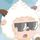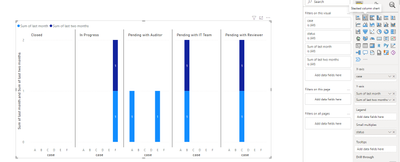cancel
Showing results for
Did you mean:Anonymous
Not applicable

## Visual to show last month status and last to last month status.

Hi All,

New to Power BI, wanted to check which visual i need to use to show last month status and last-to-last month status of the requests. Business requirement is to know the requests whose status didnt changed for the last two months, so that they can track and ask respective request owner to work on the item. The status are:

1. In Progress

2. Pending with IT Team

3. Pending with Reviewer

4. Pending with Auditor

5. Closed

2 REPLIES 2Community Support

Hi @Anonymous ,

According to your description, I made a sample and here is my solution.

Sample data:Create two columns to return status of last month and last two month.

``````status of last month =
var _a = CALCULATE(MAX('Table'[DATE]),FILTER('Table','Table'[case]=EARLIER('Table'[case])))
return IF('Table'[DATE]>= DATE(YEAR(_a),MONTH(_a)-1,DAY(_a)),'Table'[status])``````
``````status of last two months =
var _a = CALCULATE(MAX('Table'[DATE]),FILTER('Table','Table'[case]=EARLIER('Table'[case])))
return IF('Table'[DATE]>= DATE(YEAR(_a),MONTH(_a)-2,DAY(_a)),'Table'[status])``````

Then create two columns to judge whether the status changed last month(last two months). If not changed, then return 1.

``````last month =
var _a = CALCULATE(MAX('Table'[DATE]),FILTER('Table','Table'[case]=EARLIER('Table'[case])))
return
IF('Table'[DATE]=_a,IF(CALCULATE(DISTINCTCOUNTNOBLANK('Table'[status of last month]),FILTER('Table','Table'[case]=EARLIER('Table'[case])))=1,1,0))``````
``````last two months =
var _a = CALCULATE(MAX('Table'[DATE]),FILTER('Table','Table'[case]=EARLIER('Table'[case])))
return
IF('Table'[DATE]=_a,IF(CALCULATE(DISTINCTCOUNTNOBLANK('Table'[status of last two months]),FILTER('Table','Table'[case]=EARLIER('Table'[case])))=1,1,0))``````

Then you can use a stacked column chart to see clearly whose status didn't change as below.I attach my sample below for your reference.

Best Regards,
Community Support Team _ xiaosun

If this post helps, then please consider Accept it as the solution to help the other members find it more quickly.Anonymous
Not applicable

Hi,

Thank you for your response. I tried to replicate the same. However i found couple of issues, especially when all the status changes happened on same day, and for one case there is only one status.

Sample Data:Case G: has only one entry. So It should have value of last month status, but should not have value for last to last month.

Case H: How to represent if all the status changes are within one day?